# Ability to obtain and analyze the Thevenin equivalent circuit: Electronic Assignment, MMU, Malaysia

 University Multimedia University (MMU) Subject Electronic

Objective:

To characterize multiple resistive networks by its Thévenin’s equivalent circuit.

Learning Outcome:

Ability to obtain and analyze the Thevenin equivalent circuit.

Theory:

Thévenin’s Theorem: It is a process by which a complex circuit is reduced to an equivalent series circuit consisting of a single voltage source (VTH), a series resistance (RTH), and a load resistance (RL). After creating the Thévenin Equivalent Circuit, the load voltage VL or the load current IL may be easily determined.

One of the main uses of Thévenin’s theorem is the replacement of a large part of a circuit, often a complicated and uninteresting part, with a very simple equivalent. The new simpler circuit enables us to make rapid calculations of the voltage, current, and power that the original circuit can deliver to a load. It also helps us to choose the best value of this load resistance for maximum power transfer.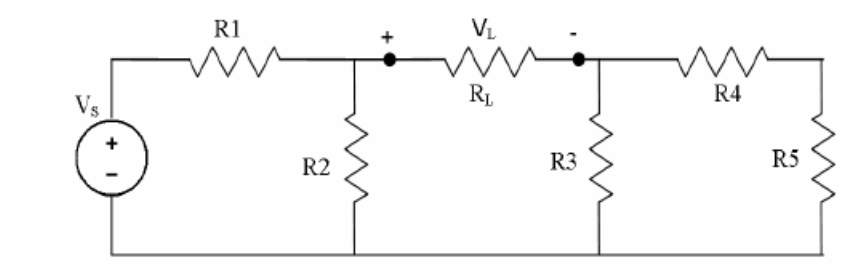Figure 7.1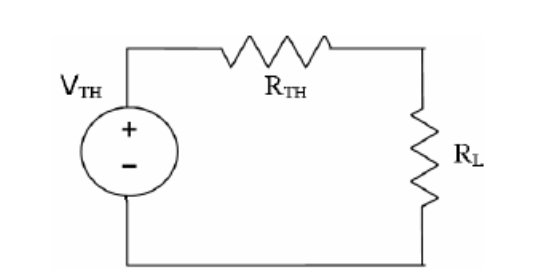Figure 7.2

Maximum Power Transfer Theorem states that an independent voltage source in series with a resistance RS or an independent current source in parallel with a resistance RS delivers maximum power to that load resistance RL for which RL = RS.

In terms of a Thévenin Equivalent Circuit, maximum power is delivered to the load resistance RL when RL is equal to the Thévenin equivalent resistance RTH of the circuit.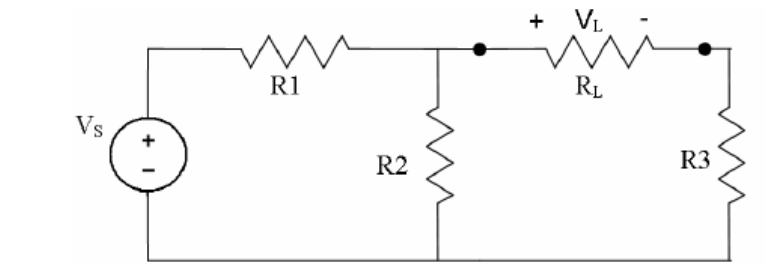Figure 7.3

Task 1: Verifying Thévenin’s theorem

• Construct the circuit of Figure 7.1 using the following component values:

R1 = 300 W

R2 = 560 W

R3 = 560 W

R4 = 300 W

R5 = 820 W

RL = 1.2 kW

VS = 10 V

• Measure the voltage VL across the load resistance, RL.

• Find VTH: Remove the load resistance RL and measure the open-circuit voltage, Voc across the terminals. This is equal to VTH.

• Find RTH: Remove the source voltage VS and replace it with a short circuit. Measure the resistance by looking into the opening where RL was with an ohmmeter. This gives RTH.

• Obtaining VTH and RTH, construct the circuit in Figure 6.2. Set the value of RTH using a variable resistor. For simulation using Proteus just use the ordinary resistor and change the value to the RTH value. Measure the VL for this circuit and compare it to the VL obtained from the circuit in Figure 7.1. State your observation.

• Construct the circuit as in Figure 7.3 using the following values:

VS = 10 V

R1 = R2 = 560 W

R3 = 820 W

RL = Variable Resistor (0-2kW)

Connect the Voltmeter across RL for measuring the load voltage (VL) and Ammeter through RL for measuring load current (IL). Vary the resistance between 600 W to 1.6 kW and note down VL and IL for each case.

## Get Help By Expert

Hire university assignment help to complete your electronic assignment. Malaysia Assignment Help has a team of professional writers who offer detailed and 100% plagiarism-free solutions on engineering assignments at a cheap price.Online Exam & Assignment Writing Services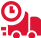51770+ Orders Delivered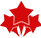4.9/5
5 Star Rating

Confidential & Secure Assignment Help For

Group Assignment Help

Online Exam -Test & Quiz

Cheapest Price Quote

Diploma & Certificate Levels

Semester & FYP Papers

Summative & Individual

GBA & Reflective

Last Minute Assistance

##### IMR652 Management Of Business Records Assignment Sample UiTM Malaysia
The process for maintaining business records should be well documented. This includes all types of documentation, such as invoices or purchase orders that are related to your company's transactions with…